# Frequency analysis and Fourier Transform¶

Faisal Qureshi
Professor
Faculty of Science
Ontario Tech University
http://vclab.science.ontariotechu.ca

## Outline¶

• Frequency analysis of images

## Jean Baptiste Joseph Fourier (1786 — 1830)¶

• Any univariate function can be re-written as a sum of sines and cosines of different frequencies (1807)
• No one believed him
• Not translated into English until 1878
• It’s true, and it is called Fourier Series

## Sine Wave¶

$$y = a \sin(\omega t + \phi)$$

Here $\omega$ represents angular frequency and $\phi$ represents phase. Recall that $t$ is time and $\omega = 2 \pi f$, where $f$ is frequency.

In :
import numpy as np
import scipy as sp
from scipy import signal
import matplotlib.pyplot as plt

In :
t = np.linspace(0, 1, 1000)

a = 1 # amplitude
f = 1 # frequency
p = 0 # phase
omega = 2 * np.pi * f # angular velocit
y = a * np.sin( omega * t + p)

plt.figure(figsize=(10, 4))
plt.title(f'Sine wave for amplitude={a}, $\omega$={f}, and $\phi$={p}')
plt.plot(t, y, 'k')
plt.xlabel('$t$')
plt.ylabel('$a \sin(2 \pi \omega t + \phi)$');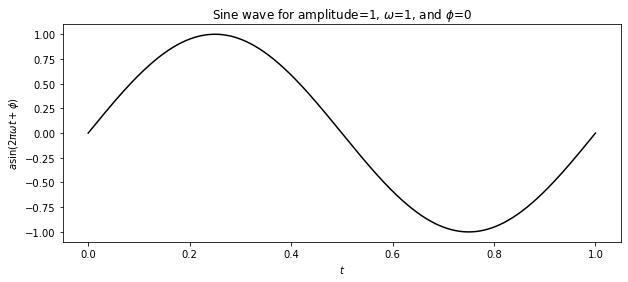In :
t = np.linspace(0, 1, 1000)

a1 = 1
f1 = 1
p1 = 0
omega1 = 2 * np.pi * f1
y1 = a1 * np.sin( omega1 * t + p1)

a2 = 0.4
f2 = 3
p2 = 0
omega2 = 2 * np.pi * f2
y2 = a2 * np.sin( omega2 * t + p2)

a3 = 1.7
f3 = 1
p3 = np.pi/2
omega3 = 2 * np.pi * f3
y3 = a3 * np.sin( omega3 * t + p3)

plt.figure(figsize=(10,4))
plt.title(f'Sine wave having different frequencies and waves')
plt.plot(t, y1, 'r-.', label=f'$a$={a1} $f$={f1}, $\phi$={p1}')
plt.plot(t, y2, 'b--', label=f'$a$={a2} $f$={f2}, $\phi$={p2}')
plt.plot(t, y3, 'k', label=f'$a$={a3} $f$={f3}, $\phi$={p3:4.3}')
plt.legend()
plt.xlabel('time')
plt.ylabel('$\sin(2 \pi \omega t + \phi)$');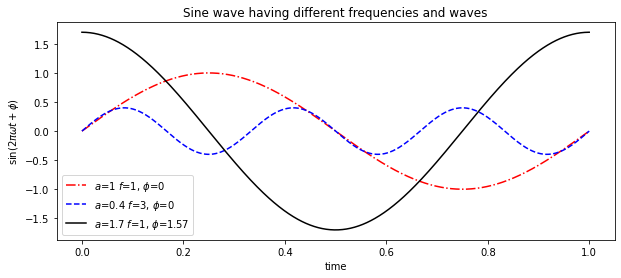## Key idea¶

• If we have enough sine waves with different amplitudes, frequencies, and phases, we can represent any signal.
In :
y = np.zeros([2, 1000])
t = np.linspace(0, 1, 1000)

a, f, p = 1, 1, 0
omega = 2 * np.pi * f
y[0,:] = a * np.sin( 2 * omega * t + p)

a, f, p = 1/3, 3, 0
omega = 2 * np.pi * f
y[1,:] = a * np.sin( 2 * omega * t + p)

plt.figure(figsize=(10,4))
plt.plot(t, y[0,:], 'r-.', label=f'$a$=1,  f={1}, $\phi$={0}', alpha=0.5)
plt.plot(t, y[1,:], 'b--', label=f'$a$=1/3,  f={3}, $\phi$={0}', alpha=0.5)
plt.plot(t, np.sum(y, 0), 'k', label='sum')
plt.legend();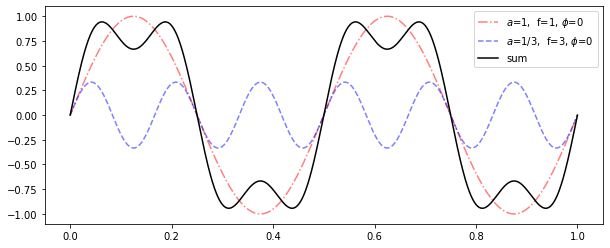## Square wave¶

We extend this idea to construct a square wave.

$$y = \sum_{f=[1,3,5,\cdots]}^\infty \frac{1}{f} \sin(2 \pi f t)$$
In :
def square_wave(n_components, n_samples=1000):
"""
Constructs a square wave using sine wave components.

Returns time (t), generated wave (y), and individual components (c)
"""

y = np.zeros([n_components, 1000])
t = np.linspace(0, 1, 1000)

a, f, p = 1, 1, 0
for i in range(n_components):
a = 1 / f
omega = 2 * np.pi * f
y[i,:] = a * np.sin( omega * t + p)
f += 2

return t, np.sum(y, 0), y

In :
n_components = 2000
t, y, c = square_wave(n_components)

plt.figure(figsize=(10,4))
for i in range(np.min([100, n_components])):
plt.plot(t, c[i,:], alpha=0.3)
plt.plot(t, y, 'k', label='sum')
plt.legend();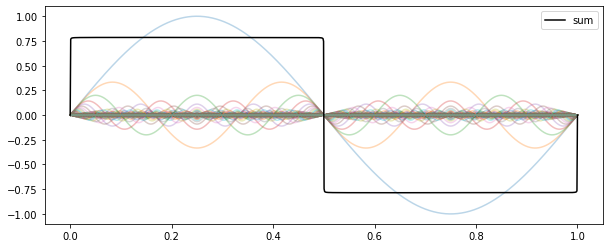## Fourier transform¶

Fourier transform often refers to both the process of decomposing a signal to its frequency components, and the resulting frequencies.

$$\mathcal{F}\{ f(x) \} = \hat{f}(\omega) = \frac{1}{2 \pi} \int_{-\infty}^\infty f(x) e^{- i \omega x} dx$$

$\hat{f}(\omega)$ refers to the Fourier transform of signal $f(x)$. We often denote Fourier transform of a function by adding a circumflex ($\hat{}$) to the symbol of that function.

Fourier transform stores the magnitude and phase at each frequency. Magnitude encodes how much signal is at a particular frequency, and phase encodes spatial information (indirectly). For mathematical convenience, this information is stored as complex numbers. Specifically at each frequency $\omega_o$, we are given

$$c + d i$$

Then signal strength (or amplitude) is

$$a_o = \pm \sqrt{c^2 + d^2}$$

and phase is

$$\phi_o = \arctan \left( \frac{d}{c} \right).$$

Recall that $i = \sqrt{-1}$.

## Complex numbers in Numpy¶

Numpy supports complex numbers. Given a complex number x

• np.complex() constructs a complex number
• x.real refers to real component
• x.imag refers to imaginary component
• np.abs(x) computes magnitude (or amplitude above)
• np.angle(x) computes angle in radians (or phase above)
In :
x = np.complex(1, 2)
print(f'complex number x    = {x}')
print(f'real part of x      = {x.real}')
print(f'imaginary part of x = {x.imag}')
print(f'magnitude of      x = {np.abs(x)}')
print(f'angle of x          = {np.angle(x)*180/np.pi} degrees')

complex number x    = (1+2j)
real part of x      = 1.0
imaginary part of x = 2.0
magnitude of      x = 2.23606797749979
angle of x          = 63.43494882292201 degrees


## Inverse Fourier Transform¶

Given a Fourier transform of a signal, it is possiible to reconstruct that signal in time domain

$$\mathcal{F}^{-1}\{ \hat{f}(\omega) \} = f(x) = \frac{1}{2 \pi} \int_{-\infty}^\infty \hat{f}(\omega) e^{i \omega x} d \omega$$

## Discrete Fourier Transform (DFT)¶

A sequence of $N$ numbers

$$x_0, x_1, x_2, \cdots, x_{N-1}$$

can be transformed into an $N$-periodic sequence of complex numbers

$$X_k = \sum_{n=0}^{N-1} x_n e^{-2 \pi k n / N}$$

Fast Fourier Transform (FFT) is $N \log N$.

It is easy to recover the original sequence $x$ given its Fourier transform $X$.

## FFT in Numpy¶

In :
n_samples = 1000

# Rate is defined as the number of samples per unit time (usually seconds).
# Notice below
frate = n_samples

n_components = 5
t, y, c = square_wave(n_components)

plt.figure(figsize=(10,4))
for i in range(np.min([100, n_components])):
plt.plot(t, c[i,:], alpha=0.3)
plt.plot(t, y, 'k', label='sum')
plt.legend();
plt.xlabel('time')
plt.title('Signal in time')

Out:
Text(0.5, 1.0, 'Signal in time')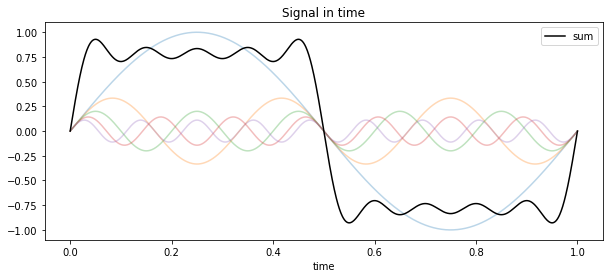Check out np.fft module for functions for performing FFT.

### Computing DFT¶

Below x denotes signal in time, and X denotes its Fourier transform.

In :
# To be consistent to mathematical notation, let x be our signal in time (seen above)
x = y

# FFT of x
X_ = np.fft.fft(x)
X = np.fft.fftshift(X_)  # fftshift shifts X such that 0 frequency sits in the center as opposed to the end


### Frequency range¶

We have 1000 samples. According to Nyquist Theorem (or Sampling Theorem), the largest frequency that we can represent is 500 Hz. As per Fourier transform theory, the transform is reflected around 0, so we also get a negative frequencies.

In :
freqs_ = np.fft.fftfreq(len(y))
freqs = np.fft.fftshift(freqs_)

print(f'Minimum frequency = {freqs.min()*1000} and Maximum frequency = {freqs.max()*1000}')

Minimum frequency = -500.0 and Maximum frequency = 499.0


### Identifying dominant frequencies¶

FFT analysis allows us to identify dominant frequencies present in the signal. Check magnitude (or amplitudes) for different frequencies, and pick frequencies that have large magnitudes.

In :
idx = np.argsort(np.abs(X[:500])) # Note that we are not sorting magnitudes, rather we sort indices using magnitudes as the decision value


Let's pick top k frequency components.

In :
k = 20
top_k_freq_idx = idx[-k:][::-1]

j = 0
freq_amp = np.empty([k, 2])
for i in top_k_freq_idx:
freq_ = np.abs(freqs[i])*frate
amp_ = np.abs(X[i])*2/frate
freq_amp[j,:] = [freq_, amp_]
#print(f'Frequency / amplitude {freq_:.3} / {amp_:4.2}')
j += 1

In :
plt.figure(figsize=(10,4))
plt.bar(freq_amp[:,0], freq_amp[:,1], width=0.1)
plt.title('Frequency components found in $x$ using FFT')
plt.xlabel('Frequencies')
plt.ylabel('Amplitudes');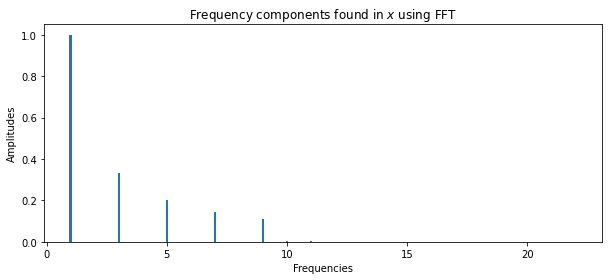## Inverse FFT¶

We can use the inverse FFT to reconstruct the original signal $x$ given its (discrete, in our case) Fourier transform $X$

In :
# Note that we are "unshifted version" of X.  The "shifted version"
# is really only useful for visualization and human understand.

# Good to remember that x_reconstructed has both a real and an imaginary part
x_reconstructed = np.fft.ifft(X_)

In :
plt.figure(figsize=(10,4))
plt.plot(t, x, 'k.', label='original', alpha=0.3)
plt.plot(t, np.real(x_reconstructed), 'r--', label='reconstructed')
plt.title('Original and reconstructed signal')
plt.legend()
plt.xlabel('time');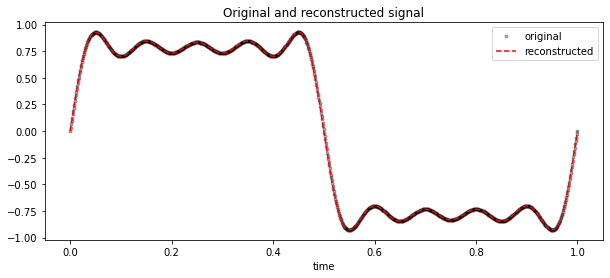## Nyquist Theorem (or Sampling theorem)¶

The Nyquist Theorem states that in order to adequately reproduce a signal it should be periodically sampled at a rate that is 2 times the highest frequency one wishes to record.

In :
t = np.linspace(0, 1, 1000)

a = 1
f = 10 # Signal frequency
p = np.pi/2
omega = 2 * np.pi * f
y = a * np.sin( omega * t + p)

plt.figure(figsize=(10, 4))
plt.title(f'Sine wave for amplitude={a}, f={f}, and $\phi$={p}')
plt.plot(t, y, 'k')
plt.xlabel('$t$')
plt.ylabel('$a \sin(2 \pi \omega t + \phi)$');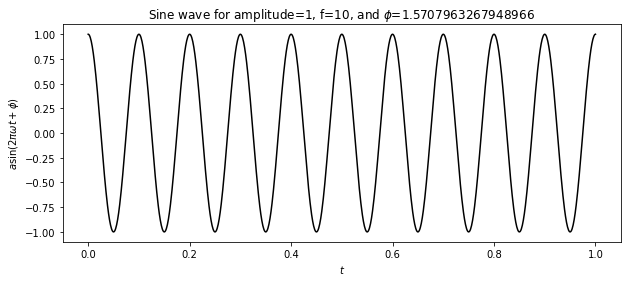### Sampling (Analog-to-Digital Conversion)¶

Sampling is perfored to perform analog-to-digital conversion.

In :
Fs = 14 # sample rate
T = 1/Fs # sampling period
sampling_duration = 1 # seconds of sampling
N = Fs * sampling_duration + 1# total points in signal (Not the 1 at the end, this is just to handle the last end point.)

t_sampled = np.arange(N)*T

In :
y_discretized = a * np.sin( omega * t_sampled + p)


#### Visualizing sampled values¶

In :
plt.figure(figsize=(10, 4))
plt.title(f'Sampled values superimposed on sine wave for amplitude={a}, $\omega$={f}, and $\phi$={p}')
plt.plot(t, y, 'k')
plt.scatter(t_sampled, y_discretized, color='red')
plt.xlabel('$t$')
plt.ylabel('$a \sin(2 \pi \omega t + \phi)$');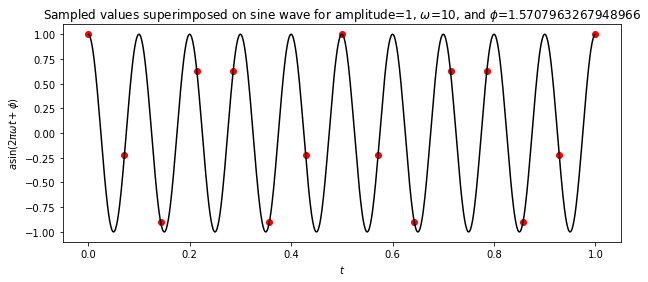### Reconstruction (Digital-to-Analog Conversion)¶

We use one of many available interpolation techniques to reconstruct the analog signal.

In :
from scipy import interpolate

method = 'previous'
method = 'cubic'
y_reconstructed_from_samples1 = interpolate.interp1d(t_sampled, y_discretized, kind=method)(t)


#### Visualizing reconstructed signal¶

In :
plt.figure(figsize=(10, 4))
plt.title(f'Sine wave for amplitude={a}, $\omega$={f}, and $\phi$={p:3.2} (interpolation method="{method}")')
plt.plot(t, y, 'k', label='Original signal', alpha=0.3)
plt.scatter(t_sampled, y_discretized, color='red', label='samples')
plt.plot(t, y_reconstructed_from_samples1, 'b', label='reconstructed from samples')
plt.xlabel('$t$')
plt.legend()
plt.ylabel('$a \sin(2 \pi \omega t + \phi)$');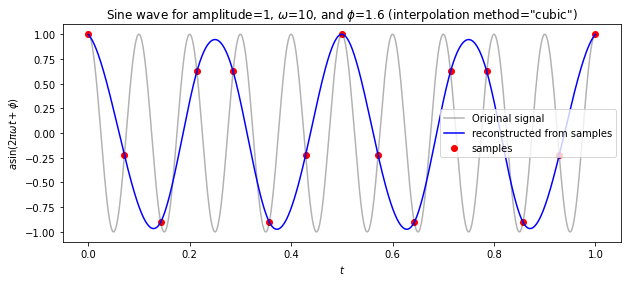## Convolution Theorem¶

Fourier transform of the convolution of two functions is the product of their Fourier transforms.

$$\mathcal{F}\{g \ast h\} = \mathcal{F}\{g\} \ast \mathcal{F}\{h\}$$

The inverse Fourier transform of the product of two Fourier transform is the convolution of the two inverse Fourier transforms.

$$\mathcal{F}^{-1}\{g h\} = \mathcal{F}^{-1}\{g\} \ast \mathcal{F}^{-1}\{h\}$$

Convolution in spatial domain is equivalent to multiplication in frequency domain.

## Properties of Fourier Transform¶

• Fourier transform of a real signal is symmetric around origin.
• The energy of the signal is the same as the energy of its Fourier transform.
• Linearity
$$\mathcal{F}\{a g(x) + b h(x)\} = a \mathcal{F}\{g(x)\} + b \mathcal{F}\{h(x)\}$$

## Fourier Transform of an Image¶

In :
import cv2 as cv

In :
img = cv.imread('data/messi.jpg', 0)

In :
plt.figure(figsize=(8,8))
plt.imshow(img, 'gray')
plt.title('Messi')
plt.xticks([])
plt.yticks([]);In :
img_fourier = np.fft.fft2(img)
img_fourier_shifted = np.fft.fftshift(img_fourier)
img_spectrum_magnitude = 20 * np.log(np.abs(img_fourier_shifted))

In :
plt.figure(figsize=(8,8))
plt.imshow(img_spectrum_magnitude, cmap = 'gray')
plt.title('Fourier transform magnitude')
plt.xticks([])
plt.yticks([]);